Next: Born Expansion Up: Scattering Theory Previous: Fundamental Equations

# Born Approximation

Equation (10.28) is not particularly useful, as it stands, because the quantity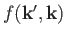depends on the unknown ket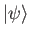. Recall that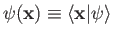is the solution of the integral equation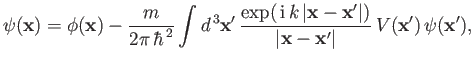(10.29)

where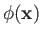is the wavefunction of the incident state. [See Equation (10.13).] According to the previous equation, the total wavefunction is a superposition of the incident wavefunction and a great many spherical waves emitted from the scattering region. The strength of the spherical wave emitted at a given point in the scattering region is proportional to the local value of the scattering potential,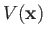, as well as the local value of the wavefunction,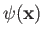.

Suppose, however, that the scattering is not particularly intense. In this case, it is reasonable to suppose that the total wavefunction,, does not differ substantially from the incident wavefunction,. Thus, we can obtain an expression forby making the substitution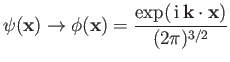(10.30)

on the right-hand side of Equation (10.29). [See Equation (10.14).] This simplification is known as the Born approximation .

The Born approximation yields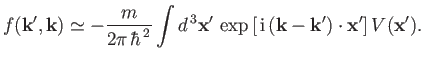(10.31)

[See Equation (10.21).] Thus,is proportional to the Fourier transform of the scattering potential,, with respect to the relative wavevector,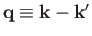.

For a spherically symmetric scattering potential,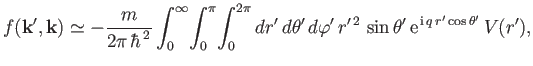(10.32)

giving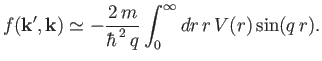(10.33)

Hence, it is clear that, for the special case of a spherically symmetric potential,depends only on the magnitude of the relative wavevector,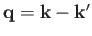, and is independent of its direction. Now, it is easily demonstrated that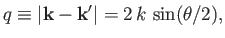(10.34)

where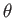is the angle subtended between the vectors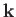and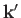. In other words,is the angle of scattering. Recall that the vectorsandhave the same length, as a consequence of energy conservation. It follows that, according to the Born approximation,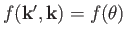for a spherically symmetric scattering potential,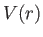. Moreover,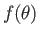is real. Finally, the differential scattering cross-section,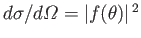, is invariant under the transformation. In other words, the pattern of scattering is identical for attractive and repulsive scattering potentials of the same strength.

Consider scattering by a Yukawa potential ,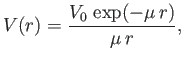(10.35)

where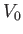is a constant, and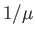measures the range'' of the potential. It follows from Equation (10.33) that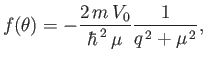(10.36)

because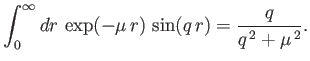(10.37)

(See Exercise 6.) Thus, the Born approximation yields a differential cross-section for scattering by a Yukawa potential of the form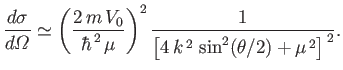(10.38)

[See Equations (10.28) and (10.34).]

The Yukawa potential reduces to the familiar Coulomb potential in the limit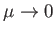, provided that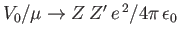. Here,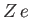and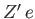are the electric charges of the two interacting particles. In the Coulomb limit, the previous Born differential cross-section transforms into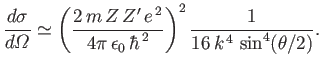(10.39)

Recalling that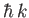is equivalent to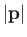, where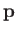is the momentum of the incident particles, the preceding equation can be rewritten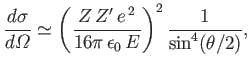(10.40)

where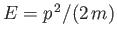is the kinetic energy of the incident particles. Equation (10.40) is identical to the well-known Rutherford scattering cross-section formula of classical physics .

The Born approximation is valid providedis not significantly different fromin the scattering region. It follows, from Equation (10.29), that the condition that must be satisfied in order that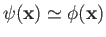in the vicinity of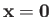is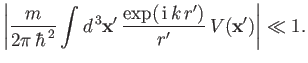(10.41)

Consider the special case of the Yukawa potential, (10.35). At low energies (i.e.,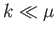), we can replace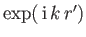by unity, giving(10.42)

as the condition for the validity of the Born approximation. Now, the criterion for the Yukawa potential to develop a bound state turns out to be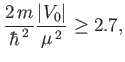(10.43)

providedis negative . Thus, if the potential is strong enough to form a bound state then the Born approximation is likely to break down. In the high-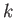limit (i.e.,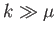), Equation (10.41) yields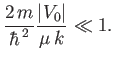(10.44)

This inequality becomes progressively easier to satisfy asincreases, implying that the Born approximation becomes more accurate at high incident particle energiesNext: Born Expansion Up: Scattering Theory Previous: Fundamental Equations
Richard Fitzpatrick 2016-01-22